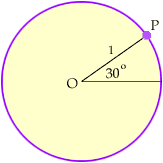ashley 10 student "My teacher wants us to find out what a unit circle is, which I found out, a circle with the radius of 1, but the problem is he wants us to show the relationship between the unit circle and the sine(30,45,60 degrees), cosine(30,45,60 degrees),and tangent ratios(30,45,60 degrees). I need help with this and my teacher will not help us out. Thanks very much ... Hi Ashley, Draw a unit circle with center at the origin O. Draw a line segment from O, making a 30o angle with the X-axis and intersecting the circle at P. What are the coordinates of P?Cheers, Penny Go to Math Central